Resource Title search

Text Search of NRICH resource titles

Or find resource begining with: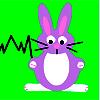Rabbit Run

Age 7 to 11 Challenge Level:

Ahmed has some wooden planks to use for three sides of a rabbit run against the shed. What quadrilaterals would he be able to make with the planks of different lengths?Rabbits in the Pen

Age 7 to 11 Challenge Level:

Using the statements, can you work out how many of each type of rabbit there are in these pens?Race Time

Age 11 to 14 Short Challenge Level:

Frank and Gabriel competed in a 200m race. Interpret the different units used for their times to work out who won.Rachel's Problem

Age 14 to 16 Challenge Level:

Is it true that $99^n$ has 2n digits and $999^n$ has 3n digits? Investigate!Racing Odds

Age 11 to 14 Challenge Level:

In a race the odds are: 2 to 1 against the rhinoceros winning and 3 to 2 against the hippopotamus winning. What are the odds against the elephant winning if the race is fair?Age 11 to 14 Short Challenge Level:

Weekly Problem 41 - 2014
Three straight lines divide an equilateral triangle into seven regions. What is the side length of the original triangle?Raffles and Strings

Age 16 to 18

How much are you likely to win from a raffle? How many loops will you make with some strings? Here, two guided examples can be found for you to work through.Rail Network

Age 7 to 11 Challenge Level:

This drawing shows the train track joining the Train Yard to all the stations labelled from A to S. Find a way for a train to call at all the stations and return to the Train Yard.Rain

Age 11 to 14 Short Challenge Level:

How many dry days did Aisha have on her holiday?Rain or Shine

Age 16 to 18 Challenge Level:

Predict future weather using the probability that tomorrow is wet given today is wet and the probability that tomorrow is wet given that today is dry.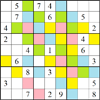Rainstorm Sudoku

Age 14 to 16 Challenge Level:

Use the clues about the shaded areas to help solve this sudokuRaising the Roof

Age 14 to 16 Challenge Level:

How far should the roof overhang to shade windows from the mid-day sun?Ramping it Up

Age 16 to 18 Challenge Level:

Look at the calculus behind the simple act of a car going over a step.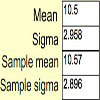Random Inequalities

Age 16 to 18 Challenge Level:

Can you build a distribution with the maximum theoretical spread?Random Pattern

Age 14 to 18 Challenge Level: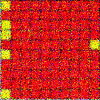Random Squares

Age 16 to 18 Challenge Level:

What is a random pattern?Random Variables - Continuous

Age 16 to 18

Resources to support an advanced course of statisticsRandom Variables - Discrete

Age 16 to 18

Resources to support an advanced course of statistics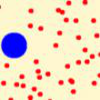Randomness and Brownian Motion

Age 16 to 18

In Classical times the Pythagorean philosophers believed that all things were made up from a specific number of tiny indivisible particles called ‘monads’. Each object contained. . . .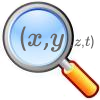Rangan's Theory of Cycles

Age 14 to 18

In this beautifully written-up investigation Abhay describes his discovery of a 'theory of cycles'.Range of Averages

Age 11 to 14 Short Challenge Level:

Find these 4 numbers, given their mode, median and range.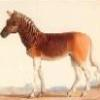Rarity

Age 16 to 18 Challenge Level:

Show that it is rare for a ratio of ratios to be rational.Rati-o

Age 11 to 14 Challenge Level:

Points P, Q, R and S each divide the sides AB, BC, CD and DA respectively in the ratio of 2 : 1. Join the points. What is the area of the parallelogram PQRS in relation to the original rectangle?Ratio and Proportion

The Ratio and Proportion collection of STEM resourcesRatio and Proportion KS2

Age 7 to 11

This collection of activities for KS2 children focuses on ratio and proportion.Ratio Cut

Age 14 to 16 Short Challenge Level:

Cutting a rectangle from a corner to a point on the opposite side splits its area in the ratio 1:2. What is the ratio of a:b?Ratio of Areas

Age 14 to 16 Short Challenge Level:

What is the ratio of the area of the hexagon to the area of the triangle?Ratio or Proportion?

Age 7 to 14

An article for teachers which discusses the differences between ratio and proportion, and invites readers to contribute their own thoughts.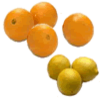Ratio Pairs 2

Age 7 to 11 Challenge Level:

A card pairing game involving knowledge of simple ratio.Ratio Pairs 3

Age 11 to 16 Challenge Level:

Match pairs of cards so that they have equivalent ratios.Ratio Riddle

Age 14 to 16 Short Challenge Level:

Can you work out the ratio b:c given the ratios a:b and a:c?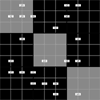Ratio Sudoku 1

Age 11 to 16 Challenge Level:

A Sudoku with clues as ratios.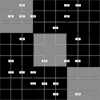Ratio Sudoku 2

Age 11 to 16 Challenge Level:

A Sudoku with clues as ratios.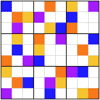Ratio Sudoku 3

Age 11 to 16 Challenge Level:

A Sudoku with clues as ratios or fractions.Ratio Swap

Age 11 to 14 Short Challenge Level:

How many adults would need to join this group of people to reverse this ratio?Ratio, Proportion & Rates of Change

Working on these problems will help your students develop a better understanding of ratio, proportion and rates of change.Ratio, Proportion & Rates of Change

Working on these problems will help you develop a better understanding of ratio, proportion and rates of change.Ratio, Proportion and Rates of Change - Short Problems

Age 11 to 16

A collection of short problems on ratio, proportion and rates of change.Rational Integer

Age 14 to 16 Short Challenge Level:

Weekly Problem 39 - 2012
For how many values of $n$ are both $n$ and $\frac{n+3}{n−1}$ integers?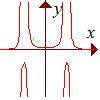Rational Request

Age 16 to 18 Challenge Level:

Can you make a curve to match my friend's requirements?Rational Roots

Age 16 to 18 Challenge Level:

Given that a, b and c are natural numbers show that if sqrt a+sqrt b is rational then it is a natural number. Extend this to 3 variables.Rational Round

Age 16 to 18 Challenge Level:

Show that there are infinitely many rational points on the unit circle and no rational points on the circle x^2+y^2=3.Rationals Between...

Age 14 to 16 Challenge Level:

What fractions can you find between the square roots of 65 and 67?Ratios and Dilutions

Age 14 to 16 Challenge Level:

Scientists often require solutions which are diluted to a particular concentration. In this problem, you can explore the mathematics of simple dilutionsRatty

Age 11 to 14 Challenge Level:

If you know the sizes of the angles marked with coloured dots in this diagram which angles can you find by calculation?Reach 100

Age 7 to 14 Challenge Level:

Choose four different digits from 1-9 and put one in each box so that the resulting four two-digit numbers add to a total of 100.Reach for Polydron

Age 16 to 18 Challenge Level:

A tetrahedron has two identical equilateral triangles faces, of side length 1 unit. The other two faces are right angled isosceles triangles. Find the exact volume of the tetrahedron.Reach for the Stars

Age 16 to 18 Challenge Level:

Some graphs grow so quickly you can reach dizzy heights...Reaction Rates

Age 16 to 18 Challenge Level:

Explore the possibilities for reaction rates versus concentrations with this non-linear differential equation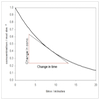Reaction Rates!

Age 16 to 18

Fancy learning a bit more about rates of reaction, but don't know where to look? Come inside and find out more...